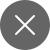# NumPy : 9 most important techniques for every Python beginner-Part 1

7+

Numpy, as the Pythoneers know, is one of the most powerful libraries of Python with a vast number of operations available, NumPy is used mostly for scientific calculations using Python. The NumPy package in Python is capable of performing high-level mathematical calculations.

You can install Numpy via pip command applicable for both MAC & Windows users:

pip install numpy

Originally, Python was not designed for numerical calculations but as it gained attention and popularity by the scientific and engineering community for its flexibility and easy to write and read code.

To begin with NumPy in Python we have to import it as a package:-

We can perform trigonometric calculations using NumPy. For example:-

We can create numpy arrays by converting lists into arrays and matrices. Numpy is very useful in matrix operations as well including transpose of a matrix and matrix multiplication.

To find unique values in a numpy array.

Numpy can also be used to find statistical tools such as mean, median and standard deviation.

To find out the maximum and minimum value using Numpy.

To save a file:-

To save a zip file:-

To load a normal file and a zip file:-

These were some of the basic operations, for more complex operations of Numpy, wait for just one day for the next part. All the operations of Numpy using Python have been performed in Jupyter Notebooks.

Stay at home, stay safe and learn Python used in Data Sciences and AI, emerge as one of the skilled Data Scientists after the lockdown and become one of the most sought after individuals as according to Harvard Business Review “Data Scientist is one of the sexiest professions of the 21st Century”.7+

#### You may also like...